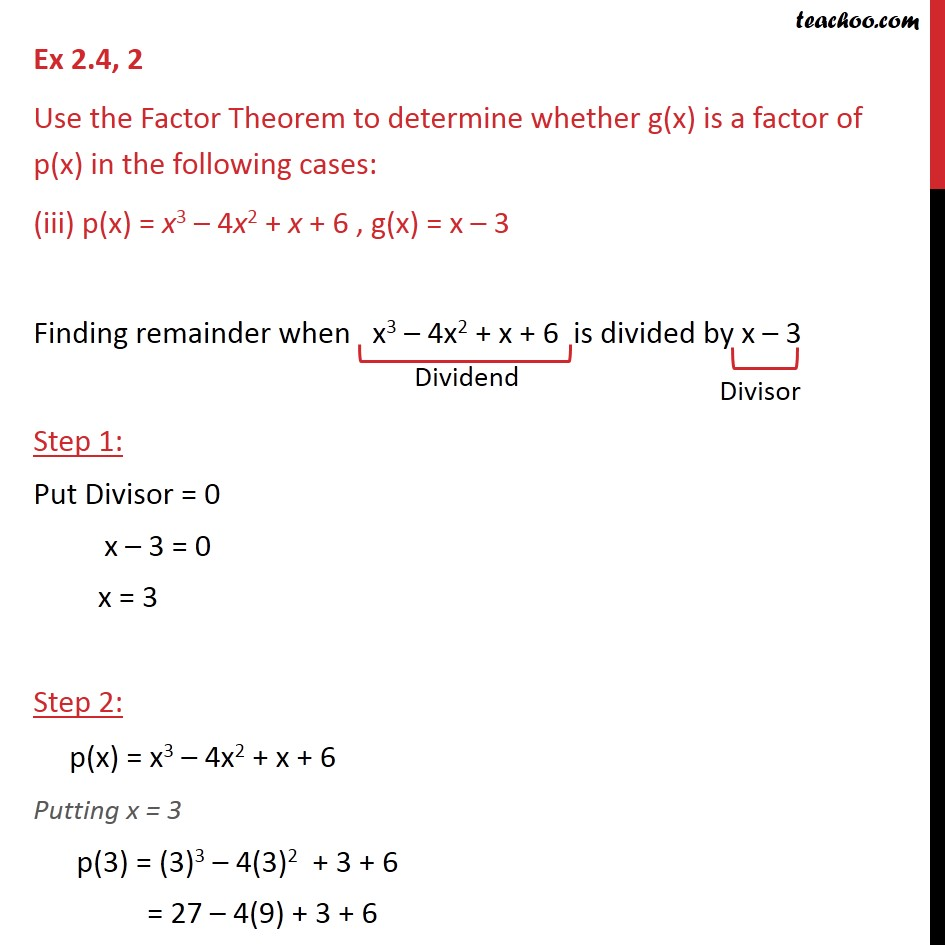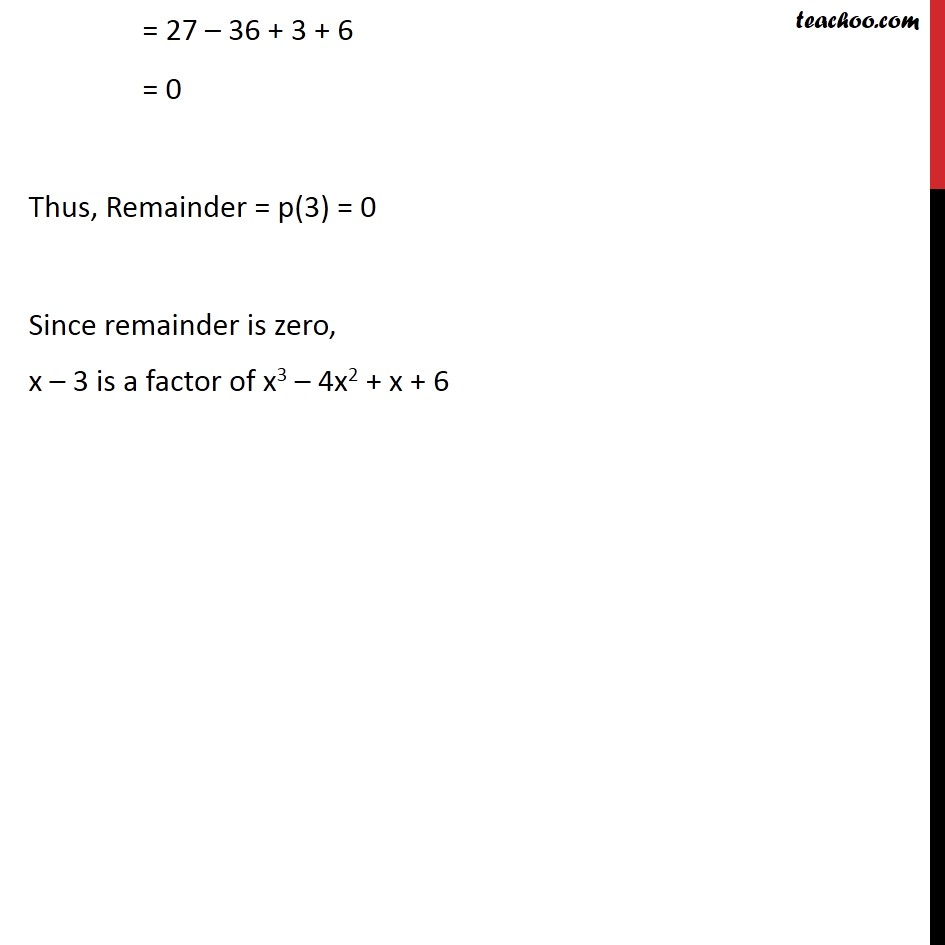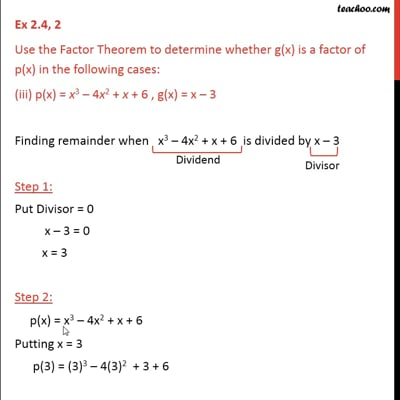Ex 2.4

Chapter 2 Class 9 Polynomials
Serial order wiseThis video is only available for Teachoo black users

Introducing your new favourite teacher - Teachoo Black, at only ₹83 per month

### Transcript

Ex 2.4, 2 Use the Factor Theorem to determine whether g(x) is a factor of p(x) in the following cases: (iii) p(x) = x3 – 4x2 + x + 6 , g(x) = x – 3 Finding remainder when x3 – 4x2 + x + 6 is divided by x – 3 Step 1: Put Divisor = 0 x – 3 = 0 x = 3 Step 2: p(x) = x3 – 4x2 + x + 6 Putting x = 3 p(3) = (3)3 – 4(3)2 + 3 + 6 = 27 – 4(9) + 3 + 6 = 27 – 36 + 3 + 6 = 0 Thus, Remainder = p(3) = 0 Since remainder is zero, x – 3 is a factor of x3 – 4x2 + x + 6